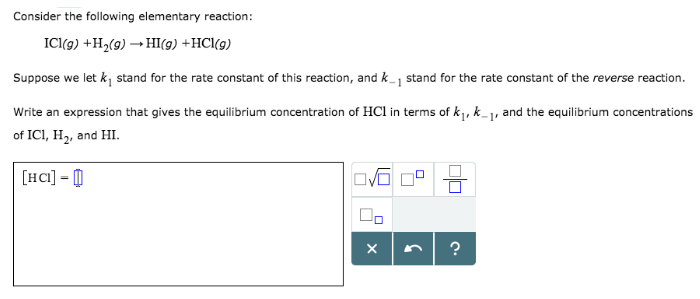# Problem: Consider the following elementary reaction: ICl (g) + H2 (g) → HI (g) + HCl (g) Suppose we let k1 stand for the rate constant of this reaction, and k-1 stand for the rate constant of the reverse reaction. Write an expression that gives the equilibrium concentration of HCl in terms of k1, k-1, and the equilibrium concentrations of ICl, H2, and HI.

###### FREE Expert Solution
82% (333 ratings)###### Problem Details

Consider the following elementary reaction:

ICl (g) + H2 (g) → HI (g) + HCl (g)

Suppose we let k1 stand for the rate constant of this reaction, and k-1 stand for the rate constant of the reverse reaction. Write an expression that gives the equilibrium concentration of HCl in terms of k1, k-1, and the equilibrium concentrations of ICl, H2, and HI.